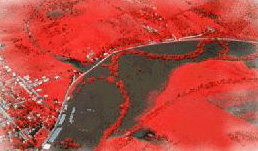Calculus on Demand at Dartmouth College Lecture 10 | Index | Lecture 12 Lecture 11## Resources

Math 3 Course Syllabus
Practice Exams

# Contents

In this lecture we continue our search for differentiation formulas by computing the derivatives of the standard trig functions.

### Quick Question

What are the three functions whose graphs are shown?### Outline

Outline for Derivatives of Trigonometric Functions

### Textbook

Derivatives of Trigonometric Functions